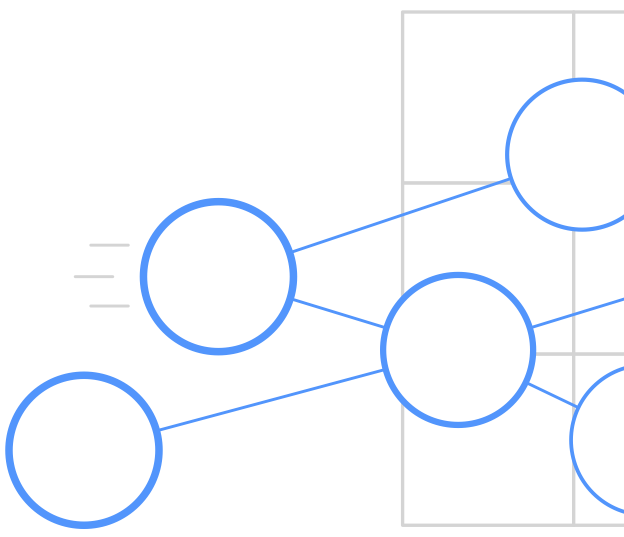#Simit

A language for computing on sparse systems

Getting Started

Language Manual

Publications

Mailing List

# The Simit C++ API

The Simit C++ API is the means for interacting with Simit. It’s design is similar to the OpenGL API, where meshes are replaced by graphs and shaders by Simit programs. The Simit C++ API consists of two main parts: a Set API for assembling graphs, and an API for loading, compiling and running Simit program on those graphs. The Simit APIs lives in the `simit::` namespace.

## Graph API (graph.h)

The Simit Graph API lets you construct graphs from sets, add elements to those sets and write data to those elements. The main class is `Set`:

``````Set points;
``````

A Simit set may be an edge set that connects other sets. The `Set` constructor can take a variable number of `Set` arguments that the resulting set connects:

``````Set springs(points, points);
``````

The next step is to add one or more field to the set. Adding a field to a set means that every element added to the set has that field. To add a field `m` that contains the mass of each spring we use the `simit::Set::addField` method:

``````FieldRef<double> m = springs.addField<double>("m");
``````

The field name `m` is given as an argument. However, you must specify the field type (a `double` per set element) using template arguments. The API also supports `bool`, `int` and `float` fields.

We can also specify vector and matrix fields using additional template arguments:

``````// Create a field with a 3-vector per set element

// Create a field with a 3x3 matrix per set element
``````

To add elements to a set you use the `Set::add` method:

``````ElementRef p1 = points.add();
``````

If the set is an edge set then you must pass the endpoints to the edge element to the `add` method:

``````ElementRef s1 = springs.add(p1, p2);
``````

To write to the field of an element you can use the `FieldRef::set` method:

``````// Set the m field of spring s
m.set(s1, 10.0);
``````

This may look very strange to C++ programmers who are used to object-oriented semantics where you write to the field of an object (`s1.m`) instead of the object of a field (`m(s1)`). However, it is consistent with the Simit way where elements belong to a set and with physical thinking where a field is defined over a system/mesh/lattice.

A more convenient syntax to set a field uses parentheses:

``````m(s1) = 10.0;
``````

You can also the field of an element you use parentheses:

``````std::cout << m.get(s1) << std::endl;
std::cout << m(s1) << std::endl;
``````

The type of accessing the field of an object is a `simit::TensorRef` (a tensor is a generalization of scalars (0 dimensions), vectors (1 dimension) and matrices (2 dimensions) to any number of dimensions. The `TensorRef` is a reference to the data of that field for that element and has the same template arguments as the field.

``````TensorRef<double> m1 = m(s1);
TensorRef<double,3> x1 = x(p1);
TensorRef<double,3,3> B1 = B(s1);
``````

You can print `TensorRef` objects like any other Simit C++ object:

``````std::cout << B1 << std::endl;
``````

To read or write a component of a non-scalar `TensorRef` use zero-indexed parentheses syntax:

``````x1(0) = 3.0;
B1(1,2) = 10.0;

std::cout << x1(0) << std::endl;    // 3.0
std::cout << B1(1,2) << std::endl;  // 10.0
``````

You can also use C++ initializer lists to set the value of a non-scalar `TensorRef`:

``````x1 = {1.0, 2.0, 3.0};
B1 = {1.0, 2.0, 3.0,
4.0, 5.0, 6.0,
7.0, 8.0, 9.0};
``````

Finally, you can iterate over the elements in a set. Note that since it is a set no order is guaranteed (including insertion order):

``````// C++ 11 foreach syntax
for (simit::ElementRef p : points) {
std::cout << p << std::endl;
}

// Iterator syntax
for (simit::Set::ElementIterator p = points.begin(); it != points.end(); ++p) {
std::cout << *p << std::endl;
}
``````

## Program API (program.h)

The Simit Program API lets you:

• Load Simit source code from strings and files into a `Program` object,
• Compile functions in the Simit source code to executable `Function` objects,
• Bind set arguments of `Function` objects to `Set` data structures, and
• Initialize and execute `Function` objects.

A `Program` represents a Simit program:

``````Program program;
``````

You can load source code into a `Program` object from a string or a file:

``````program.loadString(programString);
``````

After you have loaded source code into your `Program` object you can compile any exported function (funcs in the Simit code prefixed with the `export` modifier):

``````Function newton_method = program.compile("newton_method");
``````

A `Function` object is a runnable object (a functor). Before we execute it we must bind the arguments of the Simit function and any global `extern` variables in the Simit program to C++ data structures. The following statement binds the C++ `points` object to the `extern points` set in the Simit source code:

``````newton_method.bind("points", &points);
``````

Once all the arguments to the Simit function and all the global `extern` variables has been bound we can execute the function using the `simit::Function::runSafe` method:

``````newton_method.runSafe();
``````

The `runSafe` method lazily initializes the function if necessary, and maps/unmaps arguments between function calls. For better and more predictable performance you can manage manage this yourself. For example, if you want to execute the function 10 times with the same arguments you only have to initialize once:

``````newton_method.init();
for (int i=0; i<10; ++i) {
newton_method.unmapArgs();
newton_method.run();
newton_method.mapArgs();
}
``````

The `unmapArgs` and `mapArgs` make the bound arguments (e.g. a `Set` object) respectively unavailable and available to your C++ program. For example, if you are running your program on a GPU then `unmapArgs` will copy your data structures to GPU memory (i.e. unmap them from your local memory space) and `mapArgs` will copy the data structure back to CPU memory.